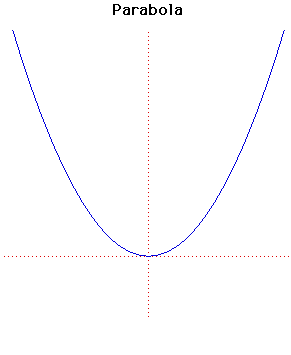# ParabolaCartesian equation:
y = ax2 + bx + c

Click below to see one of the Associated curves.

Click THIS LINK to experiment interactively with this curve and its associated curves.

The parabola was studied by Menaechmus who was a pupil of Plato and Eudoxus. He attempted to duplicate the cube, namely to find side of a cube that has a volume double that of a given cube. Hence he attempted to solve x3 = 2 by geometrical methods.

In fact the geometrical methods of ruler and compass constructions cannot solve this (but Menaechmus did not know this). Menaechmus solved it by finding the intersection of the two parabolas x2 = y and y2 = 2x.

Euclid wrote about the parabola and it was given its present name by Apollonius. The focus and directrix of a parabola were considered by Pappus.

Pascal considered the parabola as a projection of a circle and Galileo showed that projectiles follow parabolic paths.

Gregory and Newton considered the properties of a parabola which bring parallel rays of light to a focus.

The pedal of the parabola with its vertex as pedal point is a cissoid. The pedal of the parabola with its focus as pedal point is a straight line. With the foot of the directrix as pedal point it is a right strophoid (an oblique strophoid for any other point of the directrix). The pedal curve when the pedal point is the image of the focus in the directrix is a Trisectrix of Maclaurin.

The evolute of the parabola is Neile's parabola. From a point above the evolute three normals can be drawn to the parabola, while only one normal can be drawn to the parabola from a point below the evolute.

If the focus of the parabola is taken as the centre of inversion, the parabola inverts to a cardioid. If the vertex of the parabola is taken as the centre of inversion, the parabola inverts to a Cissoid of Diocles.

The caustic of the parabola with the rays perpendicular to the axis of the parabola is Tschirnhaus's Cubic.

Other Web site:

JOC/EFR/BS January 1997